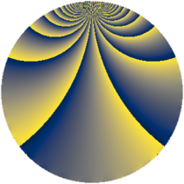# Properties

 Label 1148.2.uLevel $1148$ Weight $2$ Character orbit 1148.u Rep. character $\chi_{1148}(327,\cdot)$ Character field $\Q(\zeta_{6})$ Dimension $328$ Sturm bound $336$

# Learn more about

## Defining parameters

 Level: $$N$$ $$=$$ $$1148 = 2^{2} \cdot 7 \cdot 41$$ Weight: $$k$$ $$=$$ $$2$$ Character orbit: $$[\chi]$$ $$=$$ 1148.u (of order $$6$$ and degree $$2$$) Character conductor: $$\operatorname{cond}(\chi)$$ $$=$$ $$1148$$ Character field: $$\Q(\zeta_{6})$$ Sturm bound: $$336$$

## Dimensions

The following table gives the dimensions of various subspaces of $$M_{2}(1148, [\chi])$$.

Total New Old
Modular forms 344 344 0
Cusp forms 328 328 0
Eisenstein series 16 16 0

## Trace form

 $$328q - 2q^{2} - 2q^{4} - 12q^{5} + 4q^{8} + 152q^{9} + O(q^{10})$$ $$328q - 2q^{2} - 2q^{4} - 12q^{5} + 4q^{8} + 152q^{9} - 24q^{10} - 2q^{16} + 12q^{18} - 12q^{21} + 144q^{25} + 8q^{32} - 12q^{33} - 4q^{36} - 4q^{37} - 96q^{40} - 10q^{42} - 24q^{45} + 20q^{46} - 24q^{49} - 48q^{50} - 40q^{57} - 12q^{61} + 28q^{64} - 120q^{66} + 34q^{72} - 12q^{73} + 70q^{74} + 20q^{77} - 168q^{78} + 12q^{80} - 84q^{81} - 78q^{82} + 4q^{84} - 32q^{86} - 128q^{92} - 52q^{98} + O(q^{100})$$

## Decomposition of $$S_{2}^{\mathrm{new}}(1148, [\chi])$$ into newform subspaces

The newforms in this space have not yet been added to the LMFDB.